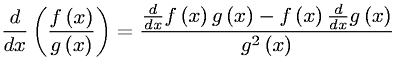Equations > Calculus > Differentiation/Differentals > Quotient Rule of Differentiation

### Quotient Rule of DifferentiationLatex Code:

MathML Code:

 $\fracd\mathrm{dx}\left(\fracf\left(x\right)g\left(x\right)\right)=\frac\fracd\mathrm{dx}f\left(x\right)g\left(x\right)-f\left(x\right)\fracd\mathrm{dx}g\left(x\right){g}^{2}\left(x\right)$

MathType 5.0: